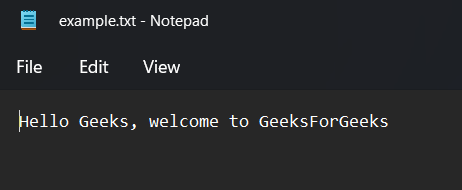GFG App
Open AppBrowser
Continue

# Copy File To Vector in C++ STL

Prerequisite:

The C++ Standard Template Library (STL) provides several useful container classes that can be used to store and manipulate data. One of the most commonly used container classes is the vector. In this article, we will discuss how to copy the contents of a file into a vector using C++ STL.

Approach:

The basic approach to copy a file into a vector is to open the file, read its contents, and store them in the vector. The C++ STL provides several functions that can be used to accomplish this task. The most commonly used functions are the ifstream class and the vector’s push_back() function. The ifstream class is used to open a file for reading. Once the file is open, we can use the >> operator to read the contents of the file one character at a time and store them in the vector. The push_back() function is used to add an element to the end of the vector.

Example:

## C++

 `// C++ Program to Copy File To Vector STL` `#include ` `#include ` `#include ` `using` `namespace` `std;`   `int` `main()` `{` `    ``// Open the file for reading` `    ``ifstream fin(``"example.txt"``);`   `    ``// Create an empty vector` `    ``vector<``char``> v;`   `    ``// Read the contents of the file and store them in the` `    ``// vector` `    ``char` `c;` `    ``while` `(fin >> c) {` `        ``v.push_back(c);` `    ``}`   `    ``// Close the file` `    ``fin.close();`   `    ``// Print the contents of the vector` `    ``for` `(``int` `i = 0; i < v.size(); i++) {` `        ``cout << v[i];` `    ``}`   `    ``return` `0;` `}`

Output:“example.txt”  fileOutput

Time complexity: O(n). // n is the number of words in the file example.txt

Auxiliary space: O(n).

In this example, the file “example.txt” is opened for reading using the ifstream class. The contents of the file are read one character at a time and stored in the vector “v” using the push_back() function. Finally, the contents of the vector are printed to the screen.

Note: Make sure that the file exists in the same directory as the code or that you provide the correct path of the file.

My Personal Notes arrow_drop_up